# Assuming that the bond dissociation energy of the C-H bond is 550.0 kJ mol-1 (131.5 kcal...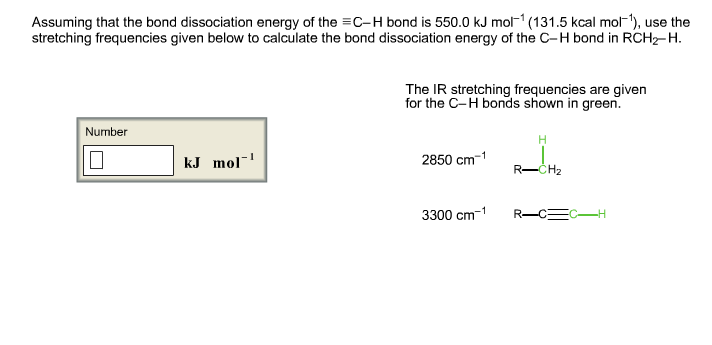Assuming that the bond dissociation energy of the C-H bond is 550.0 kJ mol-1 (131.5 kcal mol-1), use the stretching frequencies given below to calculate the bond dissociation energy of the C-H bond in RCH2-H. The IR stretching frequencies are given for the C-H bonds shown in green.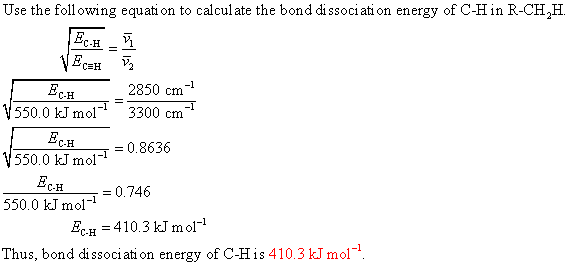#### Earn Coin

Coins can be redeemed for fabulous gifts.

Similar Homework Help Questions
• ### TABLE 6.1 BOND DISSOCIATION ENERGIES (A) OF COMMON BONDS KJ/MOL KCAL/MOL Bonds to H H2C=CH-CH2 HCC-CH,...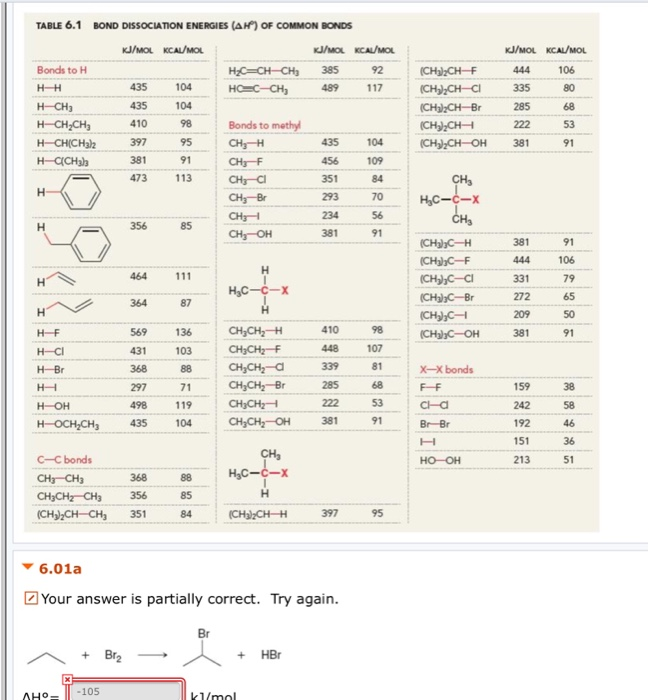TABLE 6.1 BOND DISSOCIATION ENERGIES (A) OF COMMON BONDS KJ/MOL KCAL/MOL Bonds to H H2C=CH-CH2 HCC-CH, KJ/MOL KCAL/MOL 385 92 489117 HH 435 80 104 104 KJ/MOL KCAL/MOL 444 106 335 285 222 381 91 (CH3)2CH-F (CH) CH-CI (CH3)2CHBr (CH),CH (CH3)2CH-OH H-CH3 H-CH2CH3 HCH(CH3 H- CH3) 435 104 109 Bonds to methyl CH; CHEF CH3-C1 CH3-Br CH3 CH, OH CH I I 356 85 381 91 HC-c-X CHE (CH3)3C-H (CH3),C-F (CH),C-C1 (CH3)2C-Br (CH3), (CH)C-OH 381 444 331 106 111 464...

• ### Bond Dissociation Energies (for A-B Bond broken AH, kJ/mol Bond broken → A A4, kJ/mol +...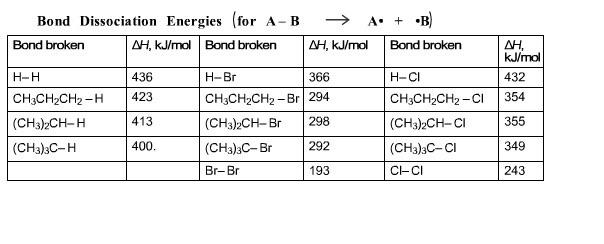Bond Dissociation Energies (for A-B Bond broken AH, kJ/mol Bond broken → A A4, kJ/mol + B) Bond broken mo H-H CH3CH2CH2-H (CH3)2CH-H (CH3)3C-H 436 423 413 400. H-Br 366 CH3CH2CH2-Br 294 (CH3)2CH-Br 298 (CH3)3C-Br292 Br-Br 193 H-CI 432 CH3CH2CH2-CI 354 (CH3)2CH-CI 355 (CH3)3C-CI 349 CI-CI 243 Alkane halogenation is a two-step reaction, as shown below. Using the table of bond dissociation energies, calculate the enthalpy of each step and the enthalpy of the overall reaction. Step 1: Number CH3CHCH3...

• ### Given the following: O2 (g) + 2F2 (g)------> 2OF2 (g) delta H=+24.5 kJ/mol Bond dissociation energies:...

Given the following: O2 (g) + 2F2 (g)------> 2OF2 (g) delta H=+24.5 kJ/mol Bond dissociation energies: F2= 159 kJ/mol; O2 (double bond) = 498 kJ/mol Calculate the bond strength of the O-F bonds in OF2.

• ### Use the table to answer questions about bond energies. Bond Bond Energy (KJ/mol) C—C 347 C=C...

Use the table to answer questions about bond energies. Bond Bond Energy (KJ/mol) C—C 347 C=C 611 C-H 414 C-O 360 C=O 736 O-O 142 O=O 498 H-O 464 (i) Calculate the bond energy for breaking all the bonds in a mole of methane, CH4 Express your answer numerically in kilojoules per mole. Change in Heat Ch4 ?=KJ/mol (ii) Calculate the bond energy for breaking all the bonds in a mole of O2 molecules? Express your answer numerically in kilojoules...

• ### The average C - H bond energy in CH4 is 415 kJ / mol. Use the following data to calculate the average C - H bond energ...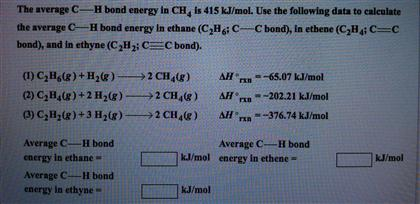The average C - H bond energy in CH4 is 415 kJ / mol. Use the following data to calculate the average C - H bond energy in ethane ( C2H6;C - C bond ) , in ethane ( C2H4;C C bond ) , and in ethyne ( C2H2;C C bond ) . C2H6 ( g ) + H2 ( g ) rightarrow 2CH4 ( g ) delta H*rxn = - 65.07 kj / mol C2H4 ( g ) +...

• ### 1. Bond Dissociation Energies and Free Radicals Bond Bond Dissociation Energies (kJ/mol) Bond BDE Bond BDE...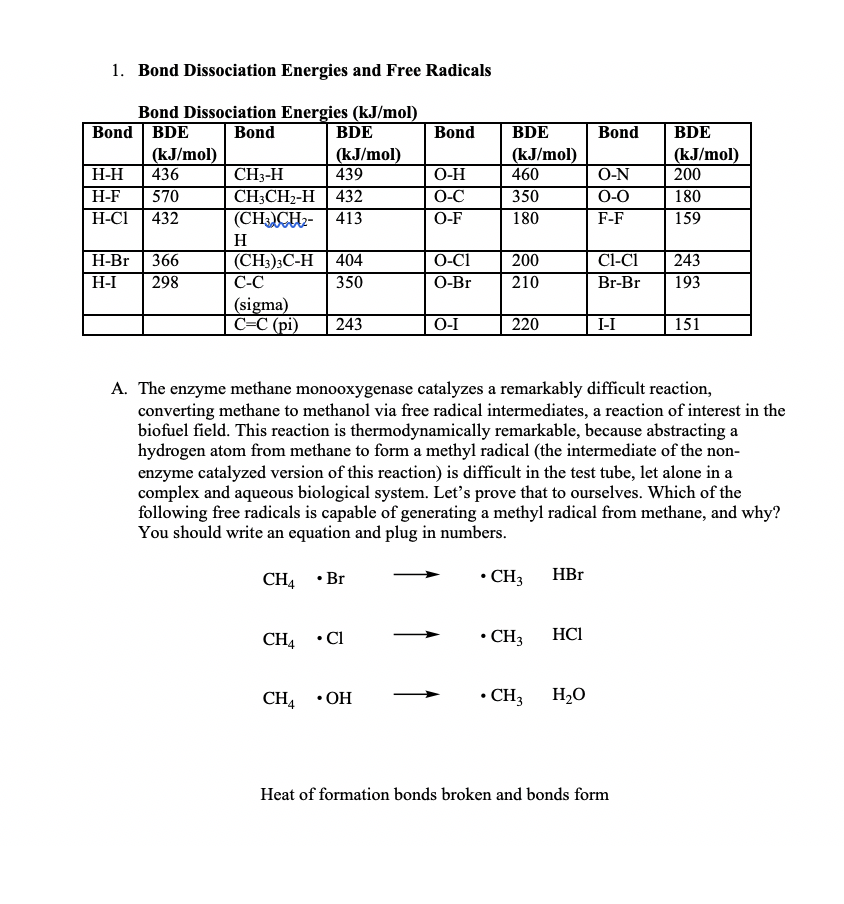1. Bond Dissociation Energies and Free Radicals Bond Bond Dissociation Energies (kJ/mol) Bond BDE Bond BDE (kJ/mol) (kJ/mol) H-H 436 CH3-H 439 H-F 570 CH3CH2-H 432 H-CI 432 (CH3CH2 413 BDE Bond (kJ/mol) 10-N 350 0-0 180 F-F O-H 0-C O-F 460 BDE (kJ/mol) | 200 180 159 | H-Br H-I 366 298 404 350 O-C1200 O-Br (CH3)3C-H C-C (sigma) C=C(pi) 210 C1-C1243 Br-Br 193 243 0 -I 220 I-I | 151 A. The enzyme methane monooxygenase catalyzes a remarkably...

• ### C-H bond BDE (kJ/mol) CH3-H 439 C2H:-H 422 (CH3)2 CH-H 414 (CH3)3 C-H 405 Table of...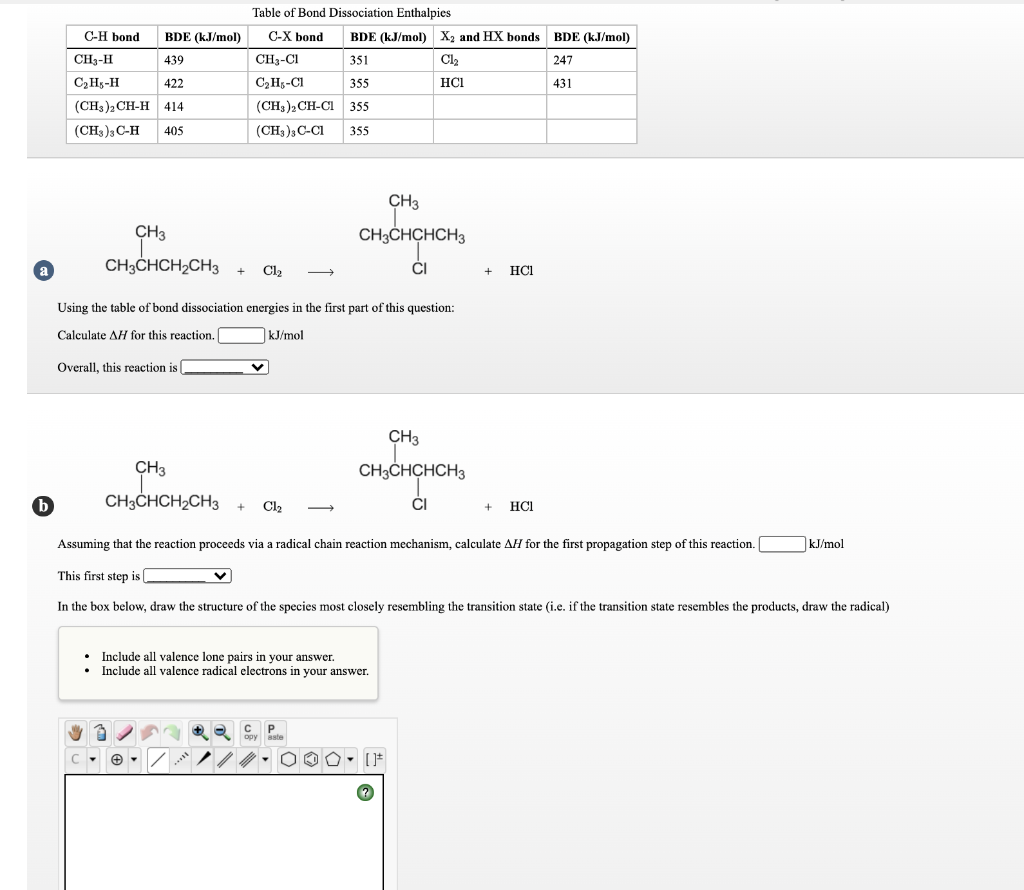C-H bond BDE (kJ/mol) CH3-H 439 C2H:-H 422 (CH3)2 CH-H 414 (CH3)3 C-H 405 Table of Bond Dissociation Enthalpies C-X bond BDE (kJ/mol) X2 and HX bonds BDE (kJ/mol) CH3-C1 351 CI 247 CH-C1 355 НСІ 431 (CH3)2CH-C 355 (CH3)3C-CI 355 CH3 CH3 CH3CHCHCH3 CH3CHCH2CH3 + a Cl2 CI HCI Using the table of bond dissociation energies in the first part of this question: Calculate AH for this reaction. kJ/mol Overall, this reaction is CH3 CH3CHCHCH3 CH3 CH3CHCH2CH3 + b...

• ### Bond N-N O=0 Bond Energy 941 kJ mol-' 499 kJ mol-' The heat of formation of...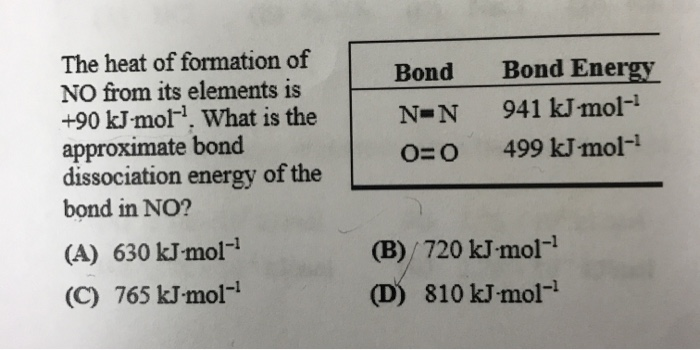Bond N-N O=0 Bond Energy 941 kJ mol-' 499 kJ mol-' The heat of formation of NO from its elements is +90 kJ mol!. What is the approximate bond dissociation energy of the bond in NO? (A) 630 kJ mol-? (C) 765 kJ mol-1 (B) 720 kJ-mol-? (D) 810 kJ mol-?

• ### Question 6 0.5 pts The table below contains the bond dissociation energies for common bonds. 410...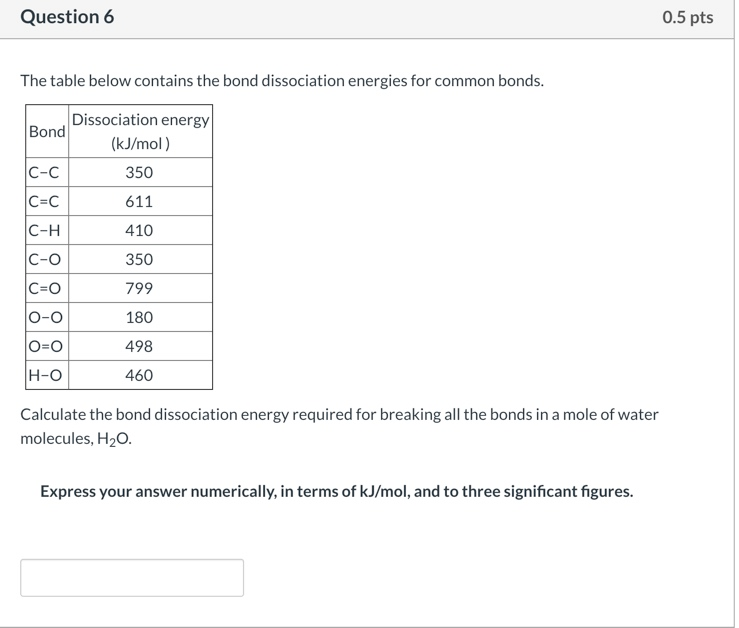Question 6 0.5 pts The table below contains the bond dissociation energies for common bonds. 410 Dissociation energy Bond (kJ/mol) |C-c 350 CNC 611 C-H 350 (c=0 799 0-0 180 O=O 498 460 C-O H-o Calculate the bond dissociation energy required for breaking all the bonds in a mole of water molecules, H20. Express your answer numerically, in terms of kJ/mol, and to three significant figures.

• ###  Question 3 3 (a) The bond dissociation enthalpies for C, and F2 are 614 kJ/mol and 154 kJ/mol respectively Usin...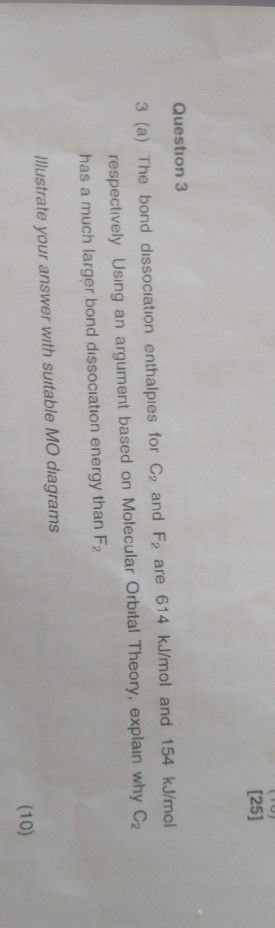Question 3 3 (a) The bond dissociation enthalpies for C, and F2 are 614 kJ/mol and 154 kJ/mol respectively Using an argument based on Molecular Orbital Theory, explain why C2 has a much larger bond dissociation energy than F2 Illustrate your answer with suitable MO diagrams (10)